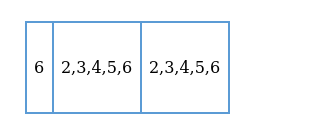# How many 3-digit numbers above 600 can be formed by using the digits

Question:

How many 3-digit numbers above 600 can be formed by using the digits 2, 3, 4, 5, 6, if repetition of digits is allowed?

Solution:

Given: We have 5 digits i.e. 2,3,4,5,6

To Find: Number of 3-digit numbers

Condition: (i) Number should be greater than 600

(ii) Repetition of digits is allowed

For forming a 3 digit number, we have to fill 3 vacant spaces.

But as the number should be above 600 , hence the first place must be occupied with 6 only because no other number is greater than 6 .

Let us represent the 3-digit numberSo the first place is filled with $6=1$ ways

Second place can be filled with 5 numbers $=5$ ways

Third place can be filled with 5 numbers $=5$ ways

Total number of ways $=1 \times 5 \times 5=25$

Total number of 3-digit numbers above 600 which can be formed by using the digits 2, $3,4,5,6$ with repetition allowed is 25